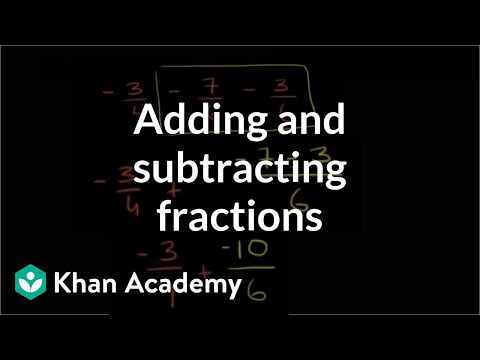Video

# Addition & subtraction: find the missing value (part 2 of 2) (Full video)

Description: Sal solves more missing value problems: 4 = -8 - __ and __ - (-2) = -7 and -4 = __ - 9. - Let's give ourselves a little bit more practice adding and subtracting positive and negative numbers. So, let's say that we have the statement, four is equal to negative eight minus blank. And, one way to think about it is, I wanna start at negative eight, so this is negative five, negative six, negative seven, negative eight.

### Other videos you might be interested in### Absolute value as distance between numbers (Full video)